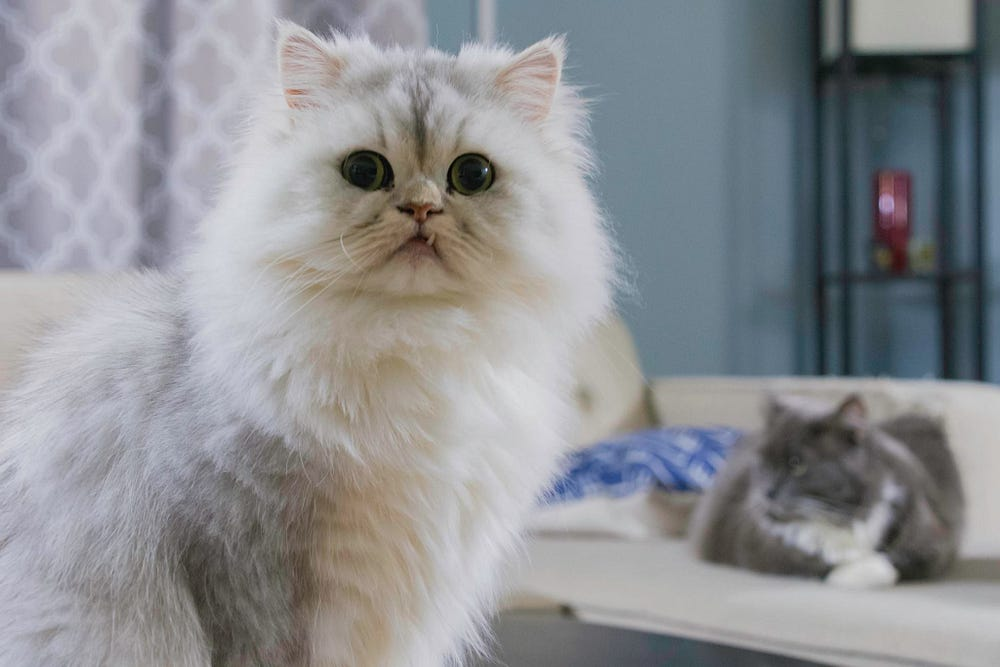# Cat Recognizer

Source: Deep Learning on Medium## Introduction

The idea for writing this article is three folds. First, to solve an interesting problem from start to end. Second, while solving the problem learn the theory, maths & intuition behind it. And third I believe, it is one of the most elegant way to get started with the entire genre of Deep Learning. For me it has to be the first step.

So, let’s dive into the problem straightaway.

## Problem Statement

You are given a dataset with the following information –

• A training set of m_train images labeled as cat (y = 1) or non-cat (y = 0)
• A test set of m_test images labeled as cat or non-cat
• Each image is of shape (num_px, num_px, 3) where height and width of the image is denoted by num_px, hence it is a square and also 3 denotes the three channels (Red, Green & Blue) or RGB in short.

You will build a simple image-recognition algorithm that can correctly classify pictures as cat or non-cat.

## Solution Approach

Here onwards we will outline the solution approach, code and discuss the concepts which are relevant to solve this problem.

Now before we go any further let me highlight why I chose this problem of recognizing cat and not cat. I could have as well chosen to classify different breeds in a cat or cat/dog/rest. The reason is very strong.

Neural Networks is a generalized class of Machine Learning algorithms. In theory it has a potential to solve any problem and that’s why it is often called as an Universal Approximation Theorem. Logistic regression is a special case of Neural Networks where it deals with binary classes like 0/1 or cat/non-cat.

The attempt is, if we understand the math, architecture and the implementation behind it, we will be able to confidently extend it to multi-class and by that we will be playing in the field of Neural Networks once and for all.

Also, in this solution we will build the code grounds-up and not use any machine learning library. Once we understand it, it will be lot easier for us to use the libraries to solve more complex problems.

Also, I will use Kaggle to share the dataset, use the Kaggle kernel (notebooks) to code it and will also encourage you all to go and create your own notebooks and play around with this dataset.

The final code will be provided in the last section of this article. Throughout the article section-wise code snippets are provided.

With this expectation, let’s get started by solving this problem.

## Step — 1: Importing required packages

We will be coding in Python, and hence we will import Python modules which we will need to solve this problem –

`import numpy as npimport matplotlib.pyplot as pltimport h5pyimport scipyfrom PIL import Imagefrom scipy import ndimage%matplotlib inline`

Instead of going over each of these modules, I will encourage you to look it up on the web if you are not aware of it. Let me pick few which are somewhat specific to this problem.

• h5py : Both our train and test set data (input data) are in .h5 format (HDF5 binary data format). The h5py package is a Pythonic interface to the HDF5. It lets you store huge amounts of numerical data, and easily manipulate that data from NumPy. For example, you can slice into multi-terabyte datasets stored on disk, as if they were real NumPy arrays. Thousands of datasets can be stored in a single file, categorized and tagged however you want.
• PIL : The Python Imaging Library (PIL) adds image processing capabilities to your Python interpreter. This library supports many file formats, and provides powerful image processing and graphics capabilities.